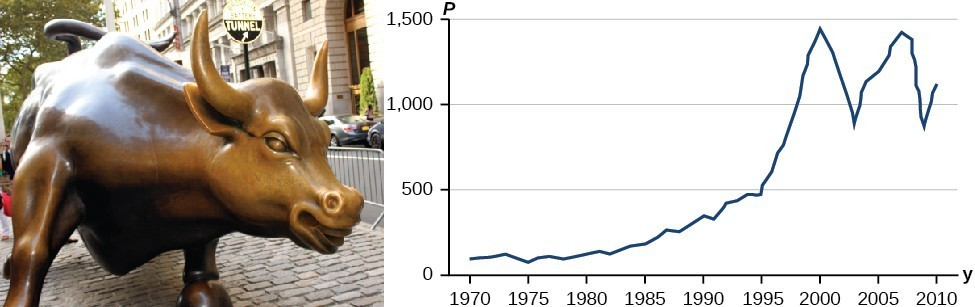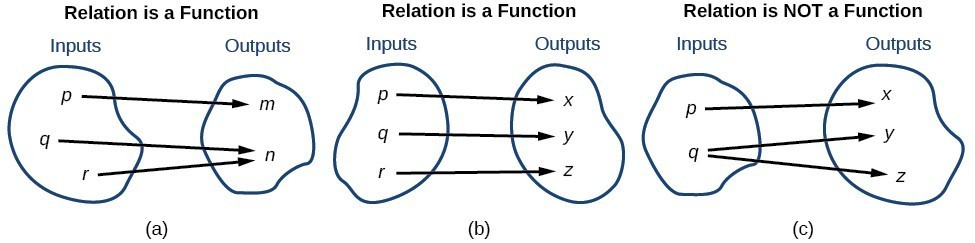## Determine whether a function is one-to-oneFigure 9

Some functions have a given output value that corresponds to two or more input values. For example, in the following stock chart the stock price was $1000 on five different dates, meaning that there were five different input values that all resulted in the same output value of$1000.

However, some functions have only one input value for each output value, as well as having only one output for each input. We call these functions one-to-one functions. As an example, consider a school that uses only letter grades and decimal equivalents, as listed in.

Letter grade Grade point average
A 4.0
B 3.0
C 2.0
D 1.0

This grading system represents a one-to-one function, because each letter input yields one particular grade point average output and each grade point average corresponds to one input letter.

To visualize this concept, let’s look again at the two simple functions sketched in (a)and (b) of Figure 10.Figure 10

The function in part (a) shows a relationship that is not a one-to-one function because inputs $q$ and $r$ both give output $n$. The function in part (b) shows a relationship that is a one-to-one function because each input is associated with a single output.

### A General Note: One-to-One Function

A one-to-one function is a function in which each output value corresponds to exactly one input value.

### Example 13: Determining Whether a Relationship Is a One-to-One Function

Is the area of a circle a function of its radius? If yes, is the function one-to-one?

### Solution

A circle of radius $r$ has a unique area measure given by $A=\pi {r}^{2}$, so for any input, $r$, there is only one output, $A$. The area is a function of radius $r$.

If the function is one-to-one, the output value, the area, must correspond to a unique input value, the radius. Any area measure $A$ is given by the formula $A=\pi {r}^{2}$. Because areas and radii are positive numbers, there is exactly one solution: $r=\sqrt{\frac{A}{\pi }}$. So the area of a circle is a one-to-one function of the circle’s radius.

### Try It 7

1. Is a balance a function of the bank account number?
2. Is a bank account number a function of the balance?
3. Is a balance a one-to-one function of the bank account number?

Solution